# APR vs. APY: What Are The Differences?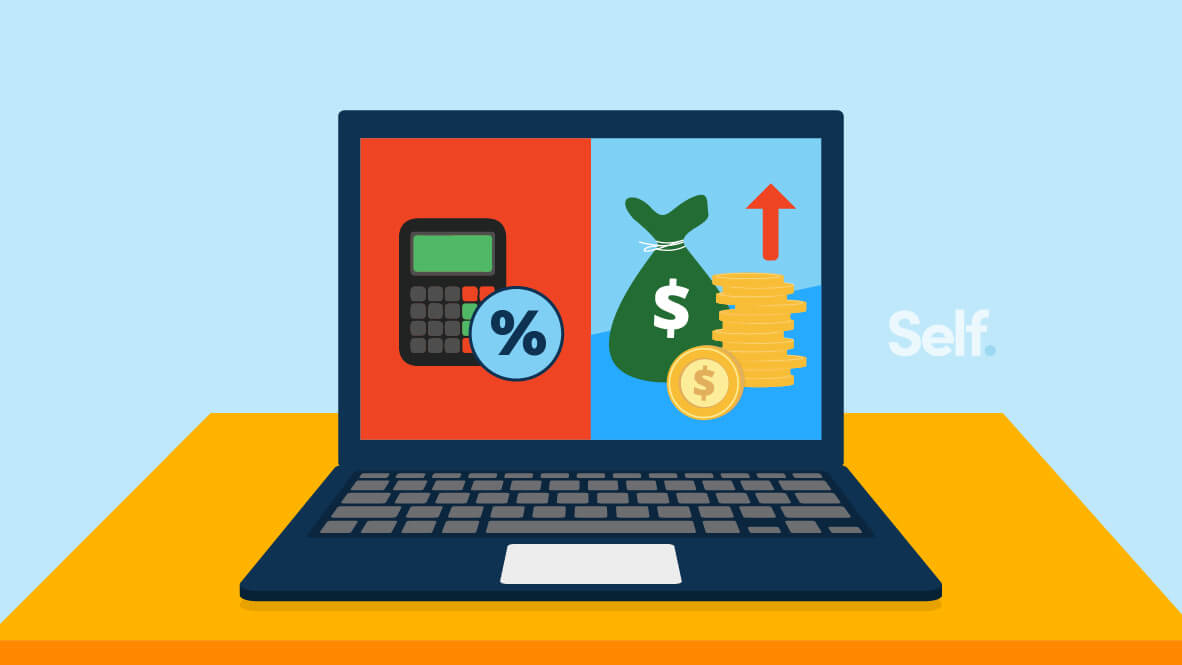By Ana Gonzalez-Ribeiro

If you have a credit card or loan, you’ve probably seen the phrase Annual Percentage Rate (APR). And if you have an interest-earning account, you may be familiar with the term Annual Percentage Yield (APY). Though they sound similar, they’re not the same. So what’s the distinction between APR vs APY? Most importantly, how do they affect you? To find out, read on.

## What is APY?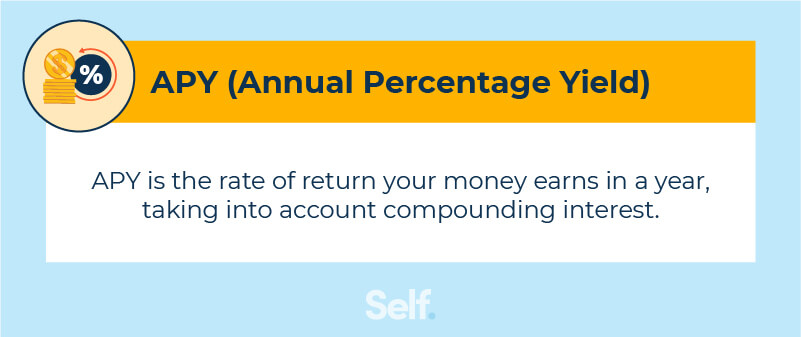APY stands for “annual percentage yield.” APY is the interest — including compounding — you receive on savings and investments in a variety of financial products and accounts.

You can earn an annual percentage yield — sometimes called “earned annual interest,” or EAR on savings accounts and money market accounts. It is also applied to investments such as certificates of deposit (CDs) and individual retirement accounts (IRAs).

## What is APR?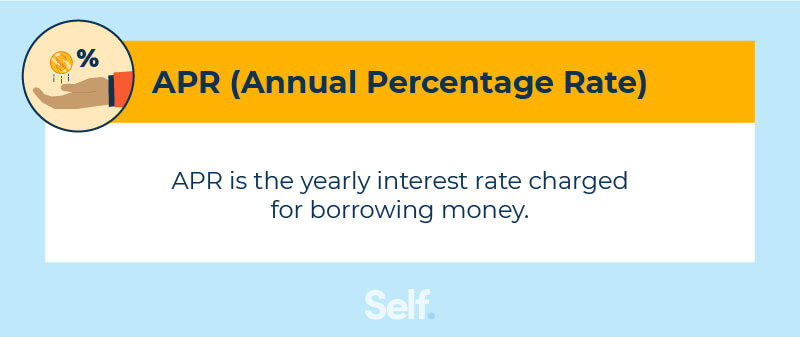APR stands for “annual percentage rate.” It is the interest rate you pay on a loan in annual terms: the yearly simple interest rate or nominal interest rate.

APR isn’t necessarily the same as your interest rate. With certain kinds of loans, APR is based on more than just the principal.

For instance, a mortgage’s APR includes the interest rate on that mortgage, the points you pay on your mortgage loan, fees, closing costs, and other charges. According to the Consumer Financial Protection Bureau, the APR on a mortgage is typically higher than the interest rate. This will affect the size of your monthly payment.

With credit cards that don’t include such fees, the APR and interest rate will closely align. It’s simply the interest you pay if you don’t pay your entire balance due each month. The CFPB defines a credit card’s interest rate as simply “the price you pay for borrowing money.”

The term APR can apply to a variety of different lending agreements, including secured loans such as car loans and home loans, as well as unsecured loans like credit cards and home equity lines of credit.

### Types of APR

Annual percentage rates are calculated based on one of two fundamentals: They may either be fixed or variable.

#### Fixed APR

Fixed APR means that the interest rate does not fluctuate. It is fixed at the same level. If any changes are made, the issuer is generally required to notify you ahead of time, and even then, adjustments will normally apply only to transactions made after you are notified. A fixed APR gives you the security of knowing that you will be paying the same rate of interest consistently, which can help with budgeting and planning.

#### Variable APR

Variable APR means the interest rate may fluctuate over time and is typically tied to an index interest rate, often the prime rate as it appears in the Wall Street Journal. A variable APR can result in you paying less if the prime rate goes down but can increase your payments if it rises.

## What are the differences between APR and APY?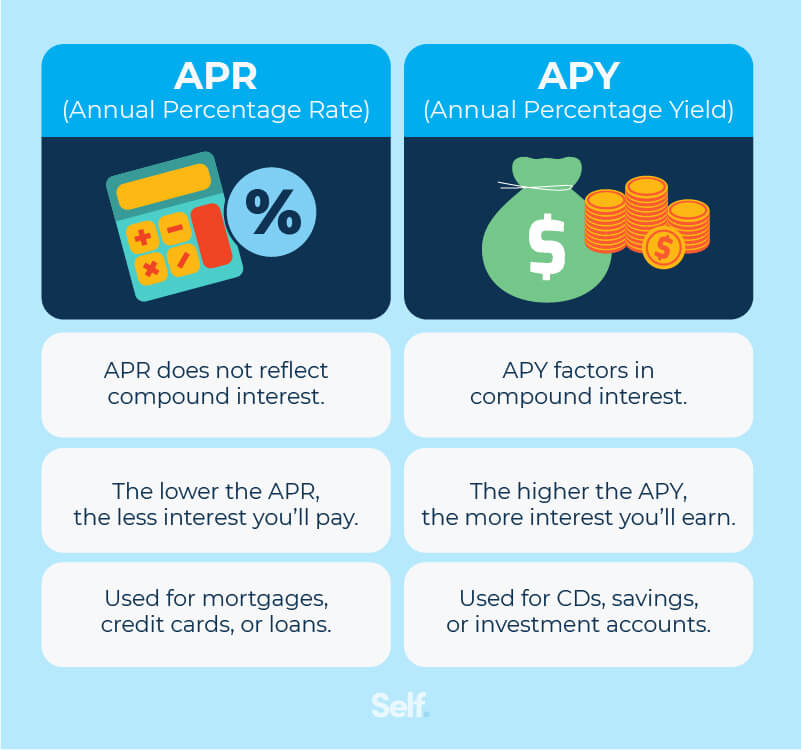The main difference between APY and APR is who gets paid: you or a lender. Other differences include what factors are taken into consideration and how each is calculated.

### Compound interest

Compound interest is calculated on a loan or deposit using two factors: The principal balance and the accumulated interest over the prior months.

As mentioned, APY takes compounding into account, while APR does not.

The frequency of compounding can vary depending on the agreement. The more often interest is compounded, the greater the yield. If interest is compounded daily, you get “interest on top of interest” every day, 365 days a year. If compounded annually, this just happens once a year. Interest can also be compounded quarterly (four times a year) or monthly (12 times a year).

If you invested \$10,000 at 5% interest, you’d have \$10,500 a year later under annual compounding, but nearly \$13 more than that if interest is compounded daily.

### Types of accounts

In most financial institutions, APY is generally used for investment products like high-yield savings accounts, CDs, and money market accounts.

APR is typically associated with credit or loan accounts. In the case of some accounts, such as mortgages, it can also include things like points and fees.

### Interest owed vs. earned

APR is the interest that you owe, while APY is the interest that you earn.

## How to calculate APR and APY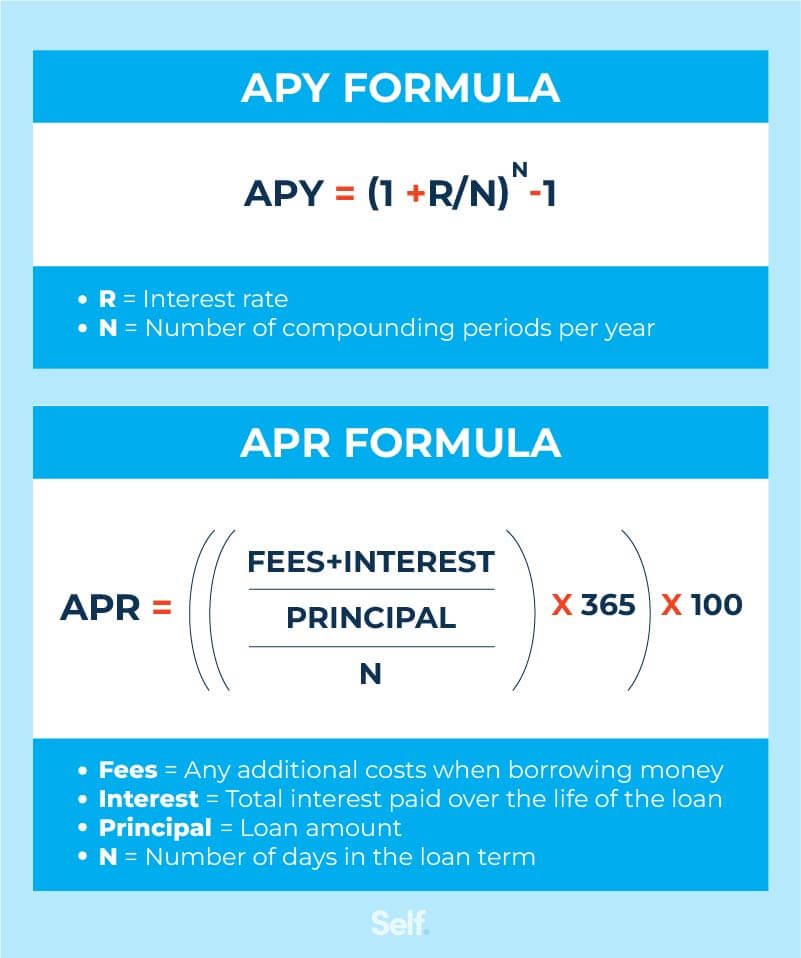If you want to know how to calculate your APR or APY, there are free online calculators you can use. Here are the formulas they use and examples.

### APR formula

To calculate APR, you will need to use this formula. We’ll walk you through it step by step.

APR = ((Fees + Interest/Principal) / Number of days in the loan term) x 365) x 100

#### APR example

For example, John takes out a \$40,000 loan to buy a car that must be paid back over the course of six years, with an interest rate of 5%, plus a \$100 origination fee to take out the loan.

1. First, calculate the Accrued amount using the formula Principal(1+(Interest rate x Length of loan)). In this case, \$40,000(1+(5% x 6) = \$52,000. Subtract the principal amount from the accrued interest to get a total interest of \$12,000.
2. Add the interest and fees: \$12,000 + \$100 = \$12,100
3. Divide this number by the principal: \$12,100 / \$40,000 = 0.3025
4. Divide again by the term of the loan: 0.3025/6 = 0.05041
5. Multiply by 100 to express as a percentage: 0.05041 x 100 = 5.041% APR

Note that if the loan term is not in full years, you’ll have to divide by the term of the loan in days and then multiply by 365 like the formula shown above.

### APY formula

To calculate APY, use the following formula:

APY = (1 + Interest rate/Annual compounding frequency)^Annual compounding frequency - 1

#### APY example

Let’s say you opened a savings account at a 6% interest rate and the interest compounds once a month.

1. First, divide the interest rate by the annual compounding frequency. Your interest compounds every month or 12 times per year: 6%/12 = 0.005
2. Add one to this number: 1 + 0.005 = 1.005
3. Multiply to the 12th power: 1.00512 = 1.0616
4. Subtract one = 0.0616 or 6.16% APY

## Comparing APR and APY

APR and APY are both ways of calculating interest payments. One reflects the cost of borrowing, and the other the yield you receive on investments.

Both affect your personal finances, and comparing how much you’re paying in APR with how much you’re receiving on your investments can help you with budgeting decisions: Is it better to focus more on paying down debt or building savings? Does a higher APY offset a high-interest credit card? What about credit utilization and your credit score?

You can be better equipped to answer these kinds of questions if you know your APR and APY.

## Sources

1. Consumer Financial Protection Bureau. “What is the difference between a mortgage interest rate and an APR?” https://www.consumerfinance.gov/ask-cfpb/what-is-the-difference-between-a-mortgage-interest-rate-and-an-apr-en-135/. Accessed December 27, 2021.
2. Experian. “What Is APR and How Does It Affect Me?”
3. https://www.experian.com/blogs/ask-experian/what-is-apr/. Accessed December 27, 2021.
4. Consumer Financial Protection Bureau. “What is a credit card interest rate? What does APR mean?” https://www.consumerfinance.gov/ask-cfpb/what-is-a-credit-card-interest-rate-what-does-apr-mean-en-44/. Accessed December 27, 2021.
5. Consumer Financial Protection Bureau. “What is the difference between a fixed APR and a variable APR?” https://www.consumerfinance.gov/ask-cfpb/what-is-the-difference-between-a-fixed-apr-and-a-variable-apr-en-45/. Accessed December 27, 2021.
6. California State Board of Equalization. “Time Value of Money - Six Functions of a Dollar,” https://www.boe.ca.gov/info/tvm/lesson9.html. Accessed December 28, 2021.
7. American Express. “APY vs APR: The Basics About How Interest is Calculated,” https://www.americanexpress.com/en-us/banking/online-savings/articles/apy-vs-apr/. Accessed December 28, 2021.